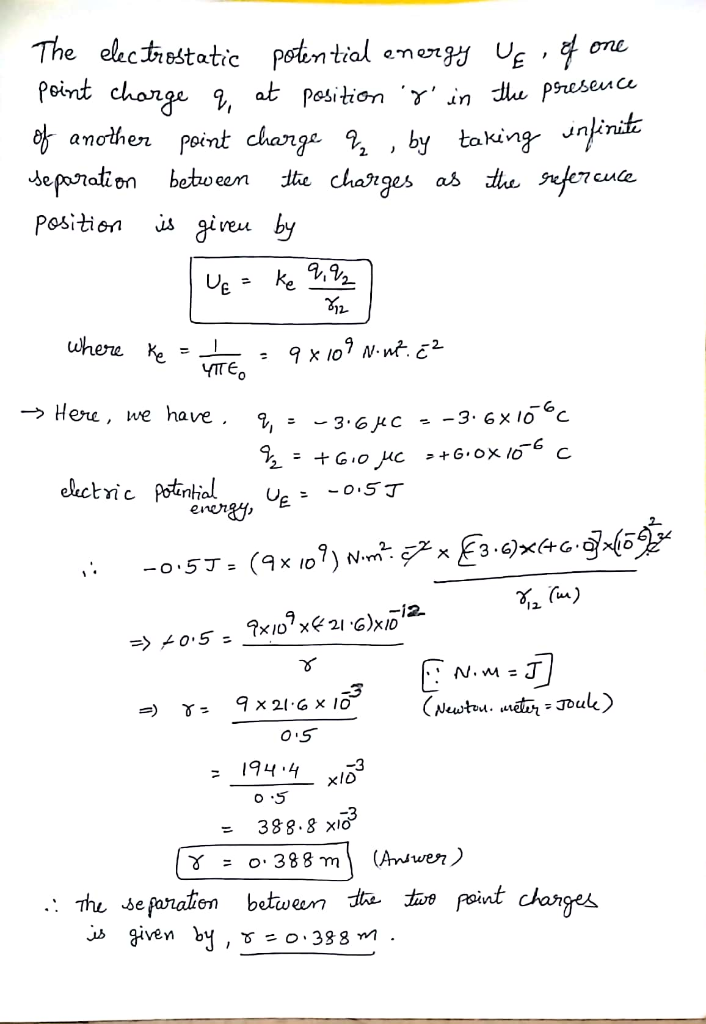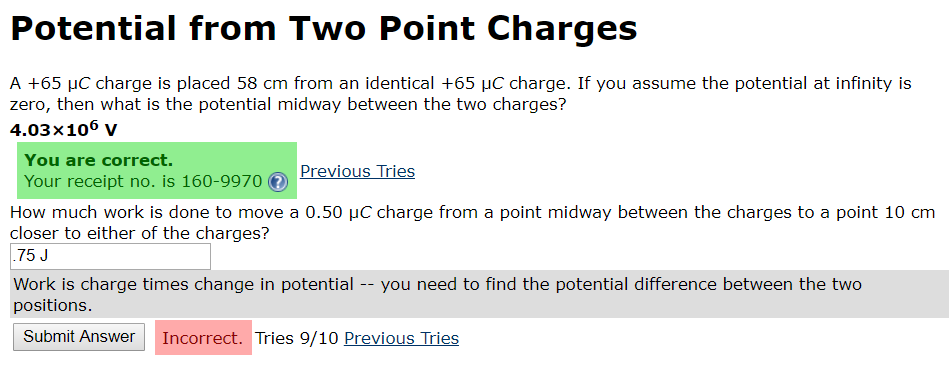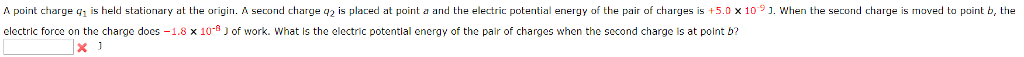Question

# How far from a - 3.6 μC point charge must a +6.0 μC point charge be...

How far from a - 3.6 μC point charge must a +6.0 μC point charge be placed for the electric potential energy of the pair of charges to be -0.5 J? (As usual, take the electric potential enregy to be zero when the charges have infinite separation.)#### Earn Coins

Coins can be redeemed for fabulous gifts.

Similar Homework Help Questions
• ### How far from a -7.40 μC point charge must a 2.50 μC point charge be placed...

How far from a -7.40 μC point charge must a 2.50 μC point charge be placed in order for the electric potential energy of the pair of charges to be -0.600 J ? (Take the energy to be zero when the charges are infinitely far apart.) Express your answer in meters

• ### 1- How far from a -7.80 μC point charge must a 2.10 μC point charge be...

1- How far from a -7.80 μC point charge must a 2.10 μC point charge be placed in order for the electric potential energy of the pair of charges to be -0.500 J ? (Take the energy to be zero when the charges are infinitely far apart.) d= ------m 2- Two stationary positive point charges, charge 1 of magnitude 3.50 nC and charge 2 of magnitude 2.00 nC , are separated by a distance of 37.0 cm . An electron...

• ### A point charge 4.60 μC is held fixed at the origin. A second point charge 1.20...

A point charge 4.60 μC is held fixed at the origin. A second point charge 1.20 μC with mass of 2.80×10−4 kg is placed on the x axis, 0.220 m from the origin. What is the electric potential energy U of the pair of charges? (Take U to be zero when the charges have infinite separation.) U U = 0.226   J   The second point charge is released from rest. What is its speed when its distance from the origin is...

• ### 1) A point charge 4.60 μC is held fixed at the origin. A second point charge...

1) A point charge 4.60 μC is held fixed at the origin. A second point charge 1.30 μC with mass of 2.80×10−4 kg is placed on the x axis, 0.260 m from the origin. A. What is the electric potential energy U of the pair of charges? (Take U to be zero when the charges have infinite separation.) B. The second point charge is released from rest. What is its speed when its distance from the origin is 0.600 m...

• ### A point charge q0 that has a charge of 0.5 μC is at the origin. (a)...

A point charge q0 that has a charge of 0.5 μC is at the origin. (a) Calculate the potential at x = 0.70 m (taking it to be zero at infinite distance). V (b) A second particle q that has a charge of 1 μC and a mass of 0.08 g is placed at x = 0.70 m. What is the potential energy of this system of charges? J (c) If the particle with charge q is released from rest,...

• ### Three equal point charges, each with charge 1.35 μC , are placed at the vertices of...

Three equal point charges, each with charge 1.35 μC , are placed at the vertices of an equilateral triangle whose sides are of length 0.250 m . What is the electric potential energy U of the system? (Take as zero the potential energy of the three charges when they are infinitely far apart.) Use ϵ0 = 8.85×10−12 C2N⋅m2 for the permittivity of free space. U = ______________ J

• ### physics

How far from a -6.20 mu {rm {rm C}} point charge must a 2.30 mu {rm {rm C}} point charge be placed in order for the electric potential energy of the pair ofcharges to be -0.300 J? (Take the energy to be zero when the charges are infinitely far apart.)

• ### A charge of 20.0-μC is held fixed at the origin. A -5.0-μC charge with a mass...

A charge of 20.0-μC is held fixed at the origin. A -5.0-μC charge with a mass of 10.0 g is launched (outwards) from the position (1.0 m, 1.0 m) at a speed of 12.0 m/s. (a) At what distance from the origin will its speed be reduced to 6.0 m/s? (b) Will it ever halt? If yes, at what distance will it halt? Three point charges are located at the corners of an equilateral triangle, whose side l = 0.5...

• ### Potential from Two Point Charges A +65 μC charge is placed 58 cm from an identical...Potential from Two Point Charges A +65 μC charge is placed 58 cm from an identical +65 μC charge. If you assume the potential at infinity is zero, then what is the potential midway between the two charges? 4.03x106 v You are correct. Previous Tries Your receipt no. is 160-9970 ( How much work is done to move a 0.50 μc charge from a point midway between the charges to a point 10 cm closer to either of the charges?...

• ### A point charge q_1 is held stationary at the origin. A second charge q_2 is placed...A point charge q_1 is held stationary at the origin. A second charge q_2 is placed at point a and the electric potential energy of the pair of charges is + 5.0 times 10^-9 J. When the second charge is moved to point b, the electric force on the charge does - 1.8 times 10^-8 J of work. What is the electric potential energy of the pair of charges when the second charge is at point b? J